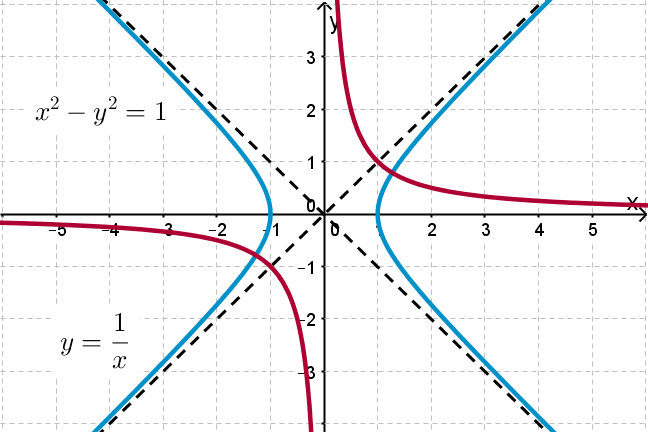## Want to keep learning?

This content is taken from the UNSW Sydney's online course, Maths for Humans: Linear, Quadratic & Inverse Relations. Join the course to learn more.
3.5

## UNSW SydneyWhat do these 2 hyperbolas have in common?

# Two classical hyperbolas in the Cartesian plane

The seemingly simple hyperbola $$\normalsize{y=1/x}$$ has many interesting aspects. It is our basic model for an inverse relation, and it embodies the fundamental arithmetical operation of taking the reciprocal of a number. It has a sister hyperbola with equation $$\normalsize{x^2-y^2=1}$$ that features prominently in Einstein’s special theory of relativity.

In this step we

• look at the geometry of these two curves

• find equations for secants and tangents for $$\normalsize{y=1/x}$$, pleasantly without calculus

## The hyperbola $$\normalsize{y=1/x}$$

Here is the graph of the deceptively simple relation $$\normalsize{y=1/x}$$, or equivalently $$\normalsize{xy=1}$$, which connects a number $$\normalsize{x}$$ to its reciprocal $$\normalsize{1/x}$$. A typical point on the curve is $$\normalsize{A=[t,1/t]}$$, such as $$\normalsize [1,1]$$, $$\normalsize [2,1/2]$$ and $$\normalsize [3,1/3]$$.Note that the equation $$\normalsize{xy=0}$$ expresses the two asymptotes $$\normalsize{y=0}$$ and $$\normalsize{x=0}$$.

## The hyperbola $$\normalsize{x^2-y^2=1}$$

Here is another hyperbola: with equation $$\normalsize{x^2-y^2=1}$$. This does not define a function in the usual sense, because it cannot be expressed as a function $$\normalsize{y=f(x)}$$. Instead this is an example of a more general relation between $$\normalsize{x}$$ and $$\normalsize{y}$$.Note that the equation $$\normalsize{x^2 - y^2 = 0}$$ expresses the two asymptotes $$\normalsize{y=x}$$ and $$\normalsize{y=-x}$$ because $$\normalsize{x^2 - y^2 = (x-y)(x+y)}$$.

Q1 (C): Can you see any similarities between the hyperbolas $$\normalsize{y=1/x}$$ and $$\normalsize{x^2-y^2=1}$$? Is there any way to get one from another?

## Finding secants to $$\normalsize{y=1/x}$$

You’ll recall that any line that intersects a curve in 2 places is called a secant line. Finding the general equation for a secant line through our hyperbola $$\normalsize{y=1/x}$$ will help us to find a general equation for a tangent line to $$\normalsize{y=1/x}$$ in the next step.

Suppose that $$\normalsize{A=[t,1/t]}$$ and $$\normalsize{B=[u,1/u]}$$ are two points on the hyperbola. To find the equation of the secant line $$\normalsize{AB}$$ we can first find the slope

$\Large{\frac{1/u-1/t}{u-t}=-\frac{1}{ut}}$

and then write the equation as

$\Large{y=-\frac{1}{ut}x + b}$

for some unknown number $$\normalsize{b}$$.

When we substitute the point $$\normalsize{A=[t,1/t]}$$ into this equation, we get

$\Large{\frac{1}{t}=-\frac{1}{u} + b}$

so that

$\Large{b= \frac{1}{t} + \frac{1}{u} }.$

It follows that the equation of the secant line is

$\Large{y=-\frac{1}{ut}x + \frac{1}{t} + \frac{1}{u} }.$

This can be rewritten also in the form

$\Large{x+tuy=t+u}.$

Q2 (E): What are the $$\normalsize{x}$$ and $$\normalsize{y}$$-intercepts of the secant line?

## Tangents to the hyperbola $$\normalsize{y=1/x}$$

A tangent line intersects a curve at only one point. So, to find the tangent line to the hyperbola at the point $$\normalsize{A=[t,1/t]}$$ we can just set $$\normalsize{t=u}$$ in the equation of the secant line. This gives

$\Large{y=-\frac{1}{t^2}x + \frac{2}{t} }$

or, equivalently

$\Large{x+t^2y=2t}.$

Hidden in this seemingly innocent derivation is a key formula of calculus: that the slope of the tangent at the point $$\normalsize A=[t,1/t]$$ is $$\normalsize - \frac{1}{t^2}$$, which is the derivative of $$\normalsize y=\frac{1}{x}$$ at the point $$\normalsize x=t$$.Q3 (E): What are the $$\normalsize{x}$$ and $$\normalsize{y}$$-intercepts of the tangent line?

Q4 (M): Show that the product of the $$\normalsize{x}$$ and $$\normalsize{y}$$-intercepts is constant, independent of the point $$\normalsize{A=[t,1/t]}$$.

Q5 (M): Show that if $$\normalsize{P}$$ is the point $$\normalsize{[2t,0]}$$ and $$\normalsize{R=[0,2/t]}$$ then $$\normalsize{Q( A,P)=Q(A,R)}$$. This verifies another of Apollonius’ claims.

A2. Setting $$\normalsize{x=0}$$ we find the $$\normalsize{y}$$-intercept is $$\normalsize{\frac{t+u}{tu}}.$$ Setting $$\normalsize{y=0}$$ we find the $$\normalsize{x}$$-intercept is $$\normalsize{t+u}.$$

A3. From the equation of the tangent line $$\normalsize{y=-\frac{1}{t^2}x + \frac{2}{t} }$$ we find that the $$\normalsize{y}$$-intercept is $$\normalsize{2/t}$$ and the $$\normalsize{x}$$-intercept is $$\normalsize{2t}$$.

A4. From the previous example we have that the intercepts are $$\normalsize{2/t}$$ and $$\normalsize{2t}$$, and so their product is $$\normalsize{2/t \times 2t= 4}$$, which is constant.

A5. The quadrance between the points $$\normalsize A=[t,1/t]$$ and $$\normalsize P=[2t,0]$$ is

$\Large Q(A,P)=(2t-t)^2+(0-\frac{1}{t})^2=t^2+\frac{1}{t^2}.$

The quadrance between the points $$\normalsize A$$ and $$\normalsize R=[0,2/t]$$ is

$\Large Q(A,R)= (0-t)^2+(\frac{2}{t}-\frac{1}{t})^2 = t^2+\frac{1}{t^2}.$

So $$\normalsize Q( A,P)=Q( A,R).$$